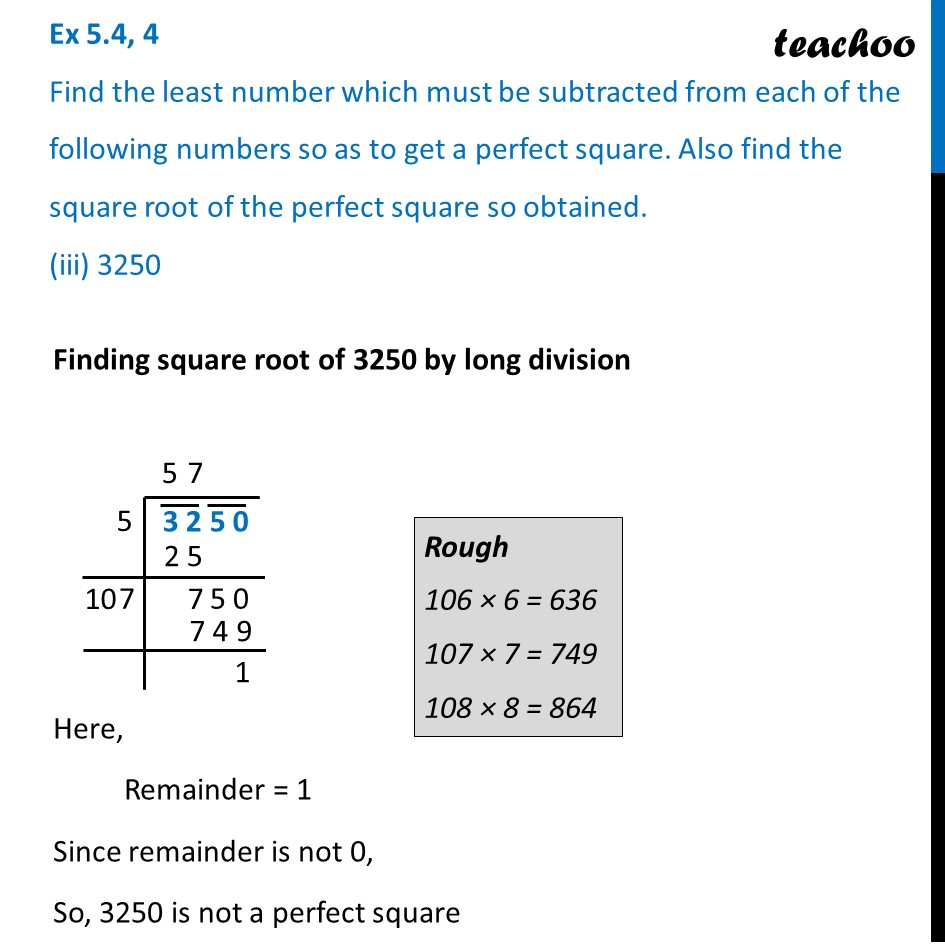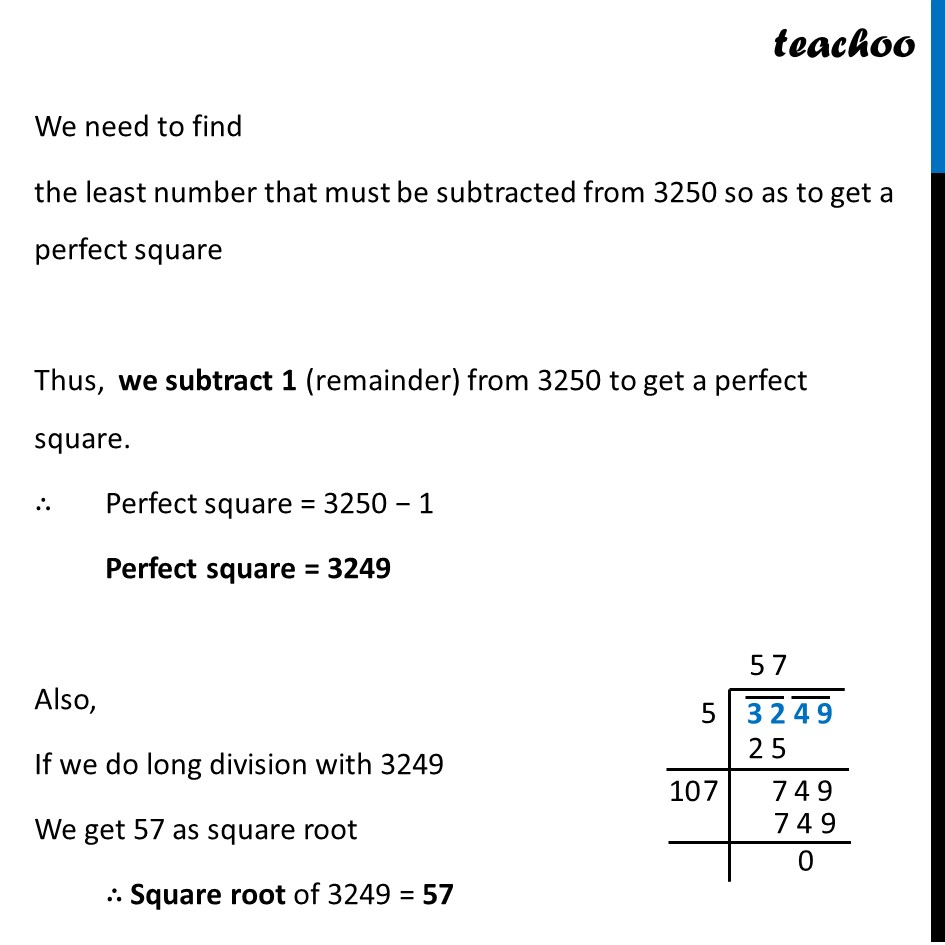Ex 5.4

Chapter 5 Class 8 Squares and Square Roots
Serial order wiseLearn in your speed, with individual attention - Teachoo Maths 1-on-1 Class

### Transcript

Ex 5.4, 4 Find the least number which must be subtracted from each of the following numbers so as to get a perfect square. Also find the square root of the perfect square so obtained. (iii) 3250 Finding square root of 3250 by long division Here, Remainder = 1 Since remainder is not 0, So, 3250 is not a perfect square Rough 106 × 6 = 636 107 × 7 = 749 108 × 8 = 864 We need to find the least number that must be subtracted from 3250 so as to get a perfect square Thus, we subtract 1 (remainder) from 3250 to get a perfect square. ∴ Perfect square = 3250 − 1 Perfect square = 3249 Also, If we do long division with 3249 We get 57 as square root ∴ Square root of 3249 = 57